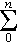﻿ The Zeros of the Partial Sums of e^z

The Zeros of the Partial Sums of ez

Roger Hui

Reference 26 of Ken Iverson’s Ph.D. thesis  was:

 Iverson, K.E., “The Zeros of the Partial Sums of ez ”, Mathematical Tables and Other Aids to Computation 7 (1953).

I quickly found a citation  on the internet:

```@Article{Iverson:1953:ZPS,
author =    "K. E. Iverson",
title =     "The Zeros of the Partial Sums of \$e^z\$",
journal =   j-MATH-TABLES-OTHER-AIDS-COMPUT,
volume =    "7",
number =    "43",
pages =     "165--168",
month =     jul,
year =      "1953",
CODEN =     "MTTCAS",
ISSN =      "0891-6837",
bibdate =   "Tue Oct 13 08:06:19 MDT 1998",
bibsource = "http://www.math.utah.edu/pub/tex/bib/
mathcomp1950.bib; JSTOR database",
acknowledgement = ack-nhfb,
fjournal =  "Mathematical Tables and Other Aids
to Computation",
}
```

From thence a helpful J Forum member provided a link to the actual text . This is the earliest Ken Iverson publication I have found so far. The paper began thus:

 The location of the zeros of certain entire functions has received considerable attention in the literature, and it was suggested by R.S. Varga that a table of the zeros of certain truncated power series might be of interest. Accordingly, the zeros of the truncated exponential series Sn(z) =zk/k! have been computed for values of n up to 23. They appear in the accompanying table with the complex zeros ordered as to modulus and with the real zero printed last.

The table entry for n = 23 stated:

 – 7.1926 8590 7451 ± 1.4750 5919 5082 i – 6.8443 1411 8665 ± 2.9355 2075 2798 i – 6.2551 3130 4907 ± 4.3657 9349 6476 i – 5.4112 0644 7035 ± 5.7481 1045 3530 i – 4.2905 2911 0822 ± 7.0608 9517 9930 i – 2.8595 2302 8914 ± 8.2762 1397 0162 i – 1.0664 4595 7935 ± 9.3553 6015 8909 i 1.1720 9815 7227 ± 10.2403 1777 0398 i 4.0025 2534 8326 ± 10.8348 6485 1671 i 7.7243 3865 4741 ± 10.9536 0414 4523 i 13.1748 6483 8907 ± 10.1262 6417 8727 i – 7.3079 8221 4646

The verb p. in J  works with polynomials. The dyad x p. y evaluates the polynomial x at y ; the monad p. x converts x between coefficients c and multiplier and roots (m;r) . The coefficients of the partial sum of ez for n=23 are:

```   c=: % ! i.24
c
1 1 0.5 0.166667 0.0416667 0.00833333 0.00138889 ...
```

Converting from coefficients to muliplier and roots:

```   mr=: p. c
mr
┌───────────┬────────────────────────────────────────────────
│3.86817e_23│13.1749j10.1263 13.1749j_10.1263 7.72434j10.9536 ...
└───────────┴────────────────────────────────────────────────
r=: > {: mr
r
13.1749j10.1263 13.1749j_10.1263 7.72434j10.9536 ...
```

The roots from 1953 are ordered in increasing modulus, with the real root last; the roots computed by J are ordered in decreasing modulus. We compare the evaluation of the roots from 1953 and of the reordered roots computed by J:

```   r1953=: _7.192685907451j1.475059195082 ...
_5 ]\ | c p. r1953
7.36698e_14 7.36698e_14 1.37043e_13 1.37043e_13 1.20857e_13
1.20857e_13 6.67108e_14 6.67108e_14 8.19945e_14 8.19945e_14
1.53714e_13 1.53714e_13 2.75251e_13 2.75251e_13 6.80251e_12
6.80251e_12 1.12317e_10 1.12317e_10   3.0922e_9   3.0922e_9
5.55512e_8  5.55512e_8 9.19177e_11           0           0

_5 ]\ | c p. 1 |. r /: | r
3.76597e_14 3.76597e_14  4.7101e_14  4.7101e_14  2.6361e_14
2.6361e_14 5.01272e_14 5.01272e_14 9.52945e_14 9.52945e_14
3.9363e_14  3.9363e_14 2.26531e_13 2.26531e_13 3.41104e_13
3.41104e_13 3.58005e_12 3.58005e_12 3.33604e_11 3.33604e_11
3.7143e_10  3.7143e_10 1.73195e_14           0           0
```

I survey the last results with considerable relief. It would have been shameful to produce a worse result 59 years later.

References

  Iverson, K.E., Machine Solutions of Linear Differential Equations — Applications to a Dynamic Economic Model, Doctoral Thesis, Harvard University, 1954-01. http://www.jsoftware.com/papers/MSLDE.htm  http://ftp.math.utah.edu/pub//tex/bib/mathcomp1950.html#Iverson:1953:ZPS  http://www.ams.org/journals/mcom/1953-07-043/ S0025-5718-1953-0057013-0/S0025-5718-1953-0057013-0.pdf  Hui, R.K.W., and Iverson, K.E., J Introduction and Dictionary, Jsoftware Inc., 2012. http://www.jsoftware.com/help/dictionary/dpdot.htm

 created: 2012-08-14 07:25 updated: 2014-01-08 14:30